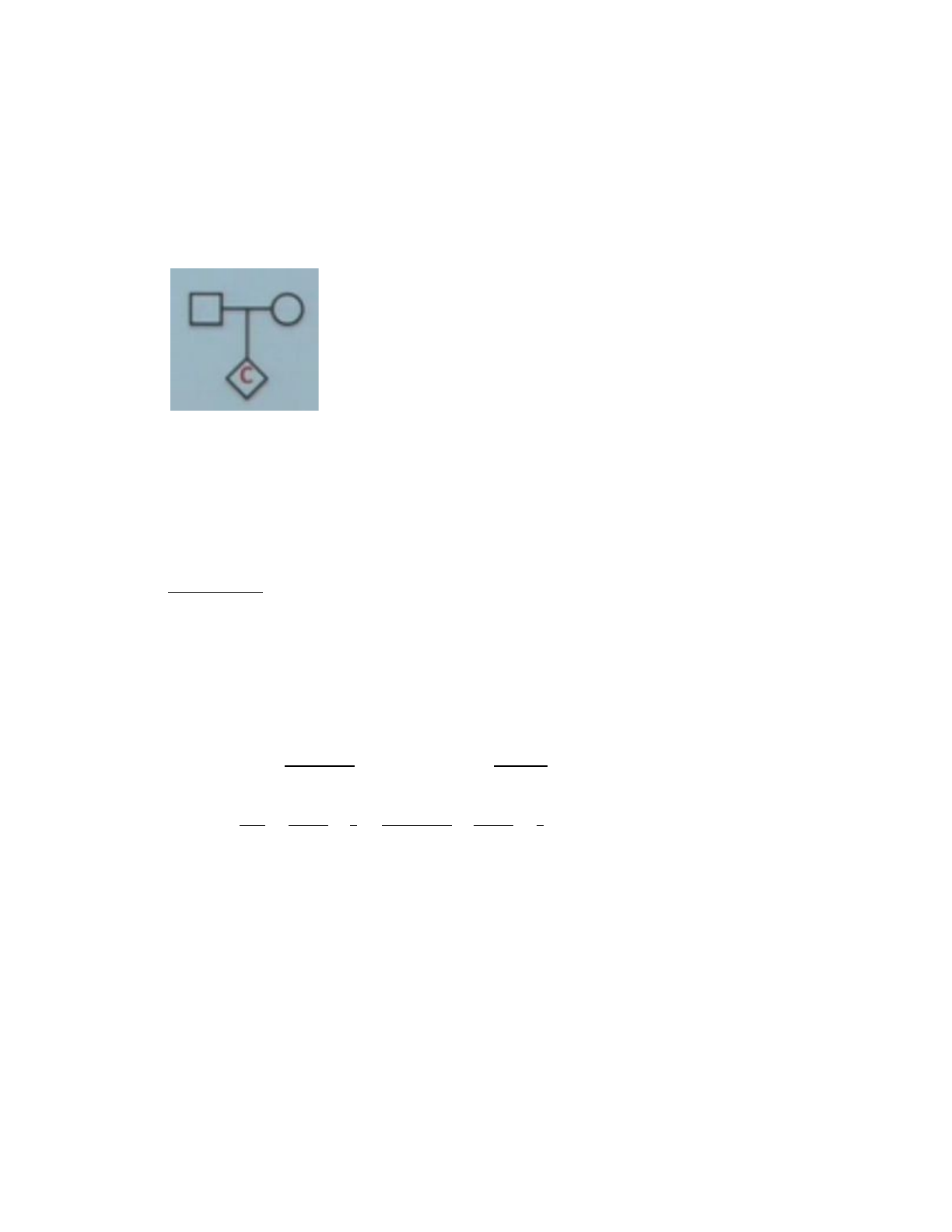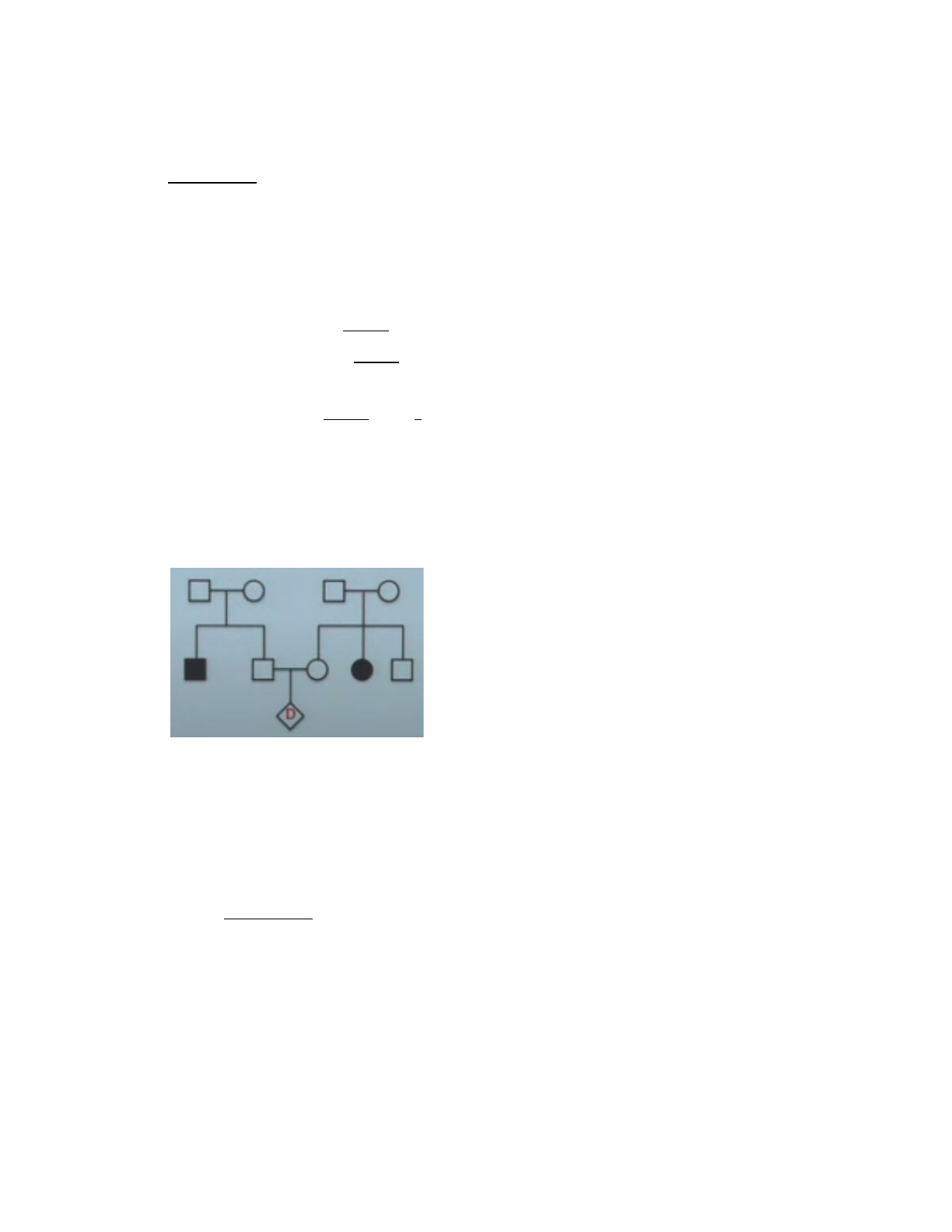# LIFESCI 4 Lecture 20: LIFESCI 4 - Lecture 20 - Review Lecture

151 views5 pages
School
Department
Course
ProfessorLIFESCI 4 - Lecture 20 - Review and Cancer
Clicker 1:
A family that lives in a population where the frequency of an autosomal recessive genetic allele
si 1 in 100 (0.01). A pedigree is shown below. What is the probability that the child will have the
disease?
What is the probability that child C will have the disease?
A) 1/100
B) 1/200
C) 1/400
D) 1/1000
E) 1/10000
Explanation:
- Population is in equilibrium
- p = frequency(a) = 1/100
- q = frequency(A) = 1 - 1/100 = 0.99
- Both parents must be heterozygous for C to have the disease
- P[C is aa] = P[Dad is Aa] X P[Mom is Aa] X ¼
- Can approximate 0.99 as 1 and 0.01 as 0
- is approximately
1−(0.01)2
2(0.99)(0.01) q
1−0
2(1)(0.01) = 2
- Same approximation for mother
- = q q /4 q
2pq
1−q2×2pq
p−2pq
2×4
1=1−(0.01)2
2(0.99)(0.01) ×2pq
p−2pq
2×4
1 2 × 2 × 1 = 2
-0.01)(0.01) 1/10000q2= ( =
Clicker 2:
Below is a pedigree from a family that lives in a population where the frequency of an
autosomal recessive genetic allele is 1 in 3 (0.33). A pedigree is shown below. What is the
probability that the child will have this disease?
What is the probability that child B will have the disease?
A) 1/4
B) 1/9
C) 1/16
Unlock document

This preview shows pages 1-2 of the document.
Unlock all 5 pages and 3 million more documents.D) 1/20
E) 1/100
Explanation:
- = frequency for (q)
- = frequency for (p)
- For child B to be homozygous recessive, both parents must be heterozygous
- P(B is aa) = P[Dad is Aa] X P[Mom is Aa] X P[both parents pass down small (a) =
¼]
- P [Dad is Aa] = (total is not since he cannot be
2pq
p + 2pq
2pq qp2+ 2 + 2q2
- P [Mom is Aa] = 2pq
p + 2pq
2
- Plug in all values for (p) and (q) into the equation
- P[B is aa] = ) /16( 2pq
p + 2pq
2
2× 4
1= 1
Clicker 3:
Below is a pedigree from a family that lives in a population where the frequency of an
autosomal recessive genetic allele is 1 in 100 (0.01)
. A pedigree is shown below. What is the
probability that the child will have this disease?
What is the probability that child D will have the disease?
A) ¼
B) 1/9
C) 1/16
D) 1/100
E) 1/300
Explanation:
- Both parents have history of disease in family
- Need to calculate probability that parents are carriers
- P(D is aa) = P[Dad is Aa] X P[Mom is Aa] X P[both parents pass down small (a) =
¼]
- P[Dad is carrier (Aa) ] =
- P[Mom is carrier (Aa) ] =
- X X ¼ = 1/9
Unlock document

This preview shows pages 1-2 of the document.
Unlock all 5 pages and 3 million more documents.

## Document Summary

Lifesci 4 - lecture 20 - review and cancer. A family that lives in a population where the frequency of an autosomal recessive genetic allele si 1 in 100 (0. 01). What is the probability that child c will have the disease: 1/100, 1/200, 1/400, 1/1000, 1/10000. Population is in equilibrium p = frequency(a) = 1/100 q = frequency(a) = 1 - 1/100 = 0. 99. Both parents must be heterozygous for c to have the disease. P[c is aa] = p[dad is aa] x p[mom is aa] x is approximately. 1 2 2 1 q. Below is a pedigree from a family that lives in a population where the frequency of an autosomal recessive genetic allele is 1 in 3 (0. 33). What is the probability that child b will have the disease: 1/4, 1/9, 1/16, 1/20, 1/100. Explanation : (cid:987) = frequency for (q) (cid:988) = frequency for (p) For child b to be homozygous recessive, both parents must be heterozygous.

## Get access

\$8 USD/m\$10 USD/m
Billed \$96 USD annuallyHomework Help
Study Guides
Textbook Solutions
Class Notes
Textbook Notes
Booster Class